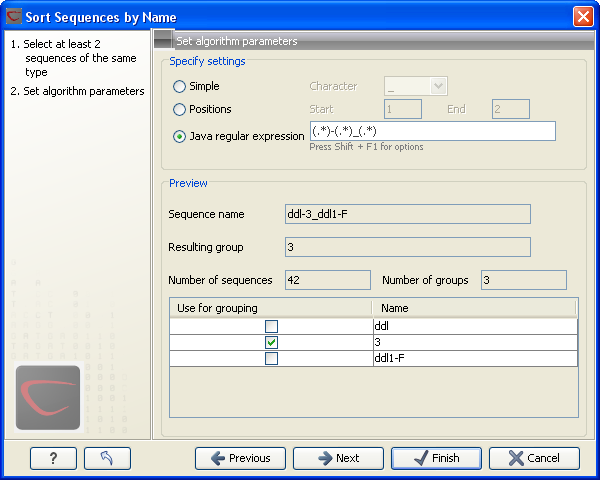### Advanced splitting using regular expressions

You can see a more detail explanation of the regular expressions syntax in Java regular expressions. In this section you will see a practical example showing how to create a regular expression. Consider a list of files as shown below:
```...
atp-29_atpA1n-F
atp-29_atpA2n-R
atp-3_atpA1n-F
atp-3_atpA2n-R
atp-66_atpA1n-F
atp-66_atpA2n-R
...
```
In this example, we wish to group the sequences into three groups based on the number after the "-" and before the "_" (i.e. 29, 3 and 66). The simple splitting as shown in figure 23.12 requires the same character before and after the text used for grouping, and since we now have both a "-" and a "_", we need to use the regular expressions instead (note that dividing by position would not work because we have both single and double digit numbers (3, 29 and 66)).

The regular expression for doing this would be `(.*)-(.*)_(.*)` as shown in figure 23.13.Figure 23.13: Dividing the sequence into three groups based on the number in the middle of the name. The round brackets () denote the part of the name that will be listed in the groups table at the bottom of the dialog. In this example we actually did not need the first and last set of brackets, so the expression could also have been `.*-(.*)_.*` in which case only one group would be listed in the table at the bottom of the dialog.